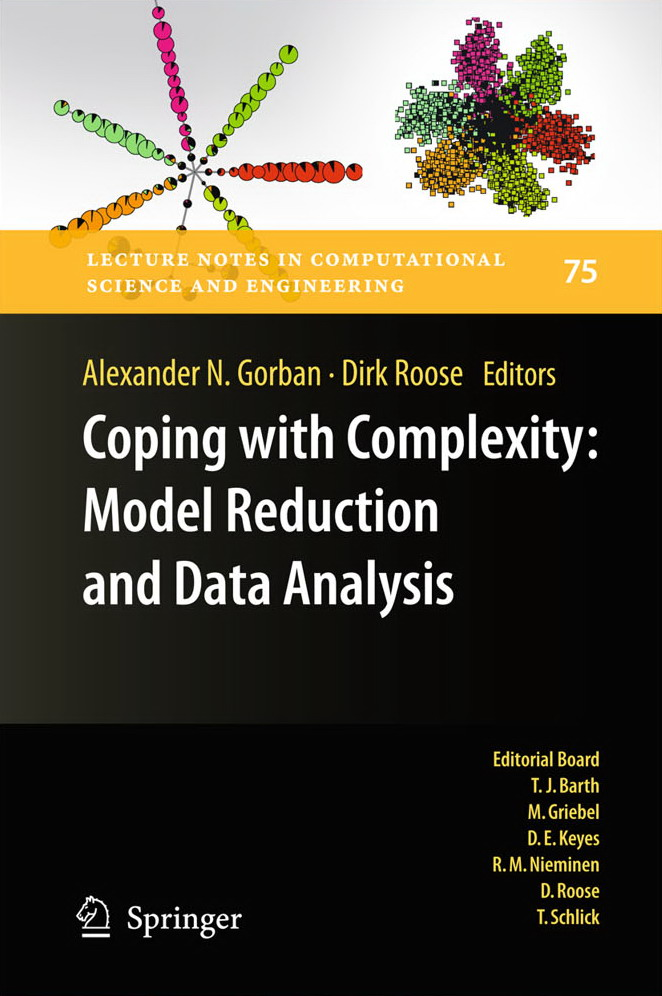• A
• A
• A
• АБB
• АБB
• АБB
• А
• А
• А
• А
• А
Обычная версия сайта

## Universal Algorithms, Mathematics of Semirings and Parallel Computations

P. 63-89.
Litvinov G., Maslov V., Rodionov A., Sobolevski A.

This is a survey paper on applications of mathematics of semirings to numerical analysis and computing. Concepts of universal algorithm and generic program are discussed. Relations between these concepts and mathematics of semirings are examined. A very brief introduction to mathematics of semirings (including idempotent and tropical mathematics) is presented. Concrete applications to optimization problems, idempotent linear algebra and interval analysis are indicated. It is known that some nonlinear problems (and especially optimization problems) become linear over appropriate semirings with idempotent addition (the so-called idempotent superposition principle). This linearity over semirings is convenient for parallel computations.

### В книгеIss. 75. Berlin; Heidelberg: Springer, 2011.